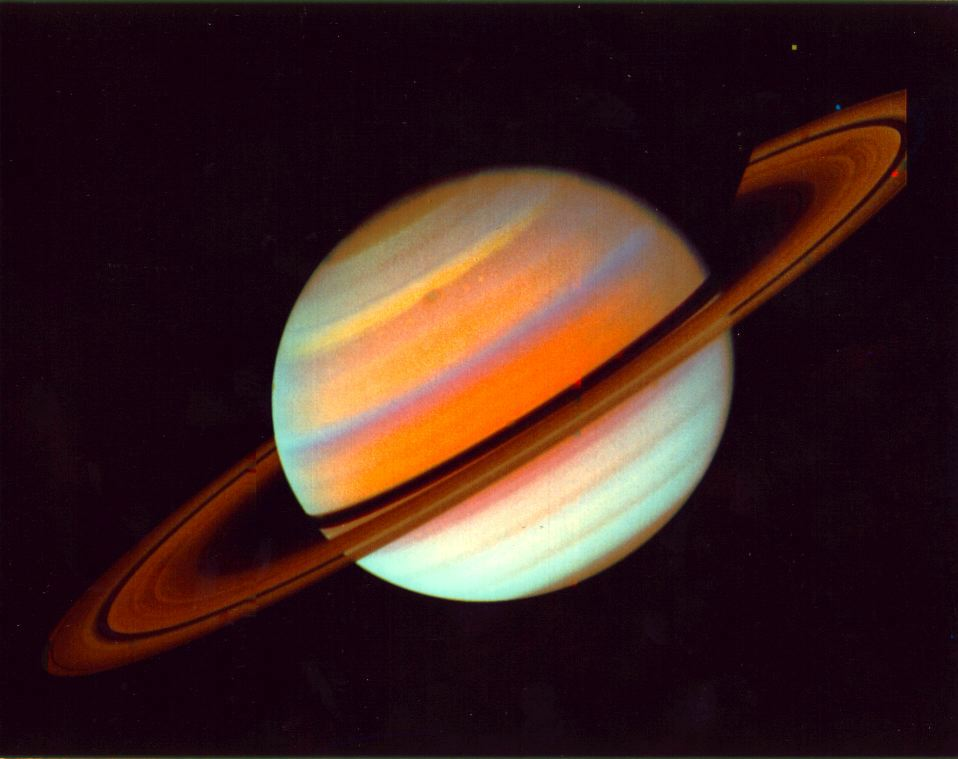# If you weighed 100 pounds on Earth, how much would you weigh on Saturn?

The simple answer is that taken as an average, your weight on Saturn would be your own body weight, multiplied by 1.08. Therefore, if you weigh 100 pounds on Earth, you would weigh 108 pounds on Saturn.

The Effects of Gravitational Acceleration on Weight

Gravitational acceleration is the effect that mass has on an object's ability to attract other objects through gravitational force. The greater the mass of an object, the more gravitational acceleration it creates.This is why you would only weigh a sixth of your body weight on the Moon - you would still have the same mass, since all the atoms in your body would still be there, but the gravitational force that the Moon is able to exert upon you is far lower, because it is so much smaller than the Earth.

However, Saturn has 95 times the mass of Earth, so it has the force to crush your body completely, depending upon where you stand!

If you were to fly through the upper atmosphere of Saturn, the gravitational acceleration would be roughly equivalent to that of standing on the surface of the Earth - so you'd weigh about the same too.

If however, by some miracle, you were able to make it down to the liquid surface of Saturn, gravitational acceleration there would be about 1.139 times that of Earth. Therefore, you'd weigh almost 14% more on Saturn at this point.
1 Person thanked the writer.